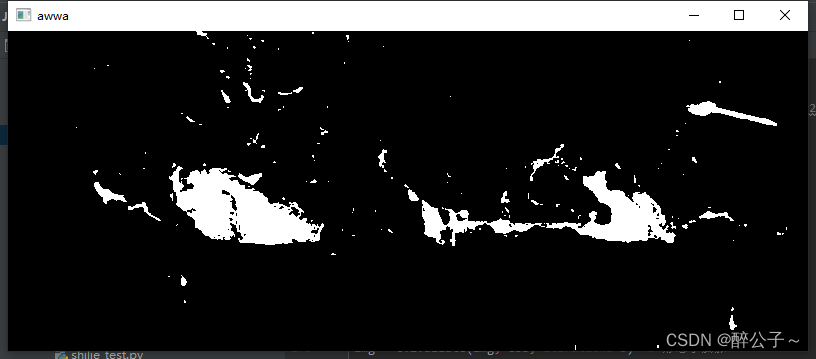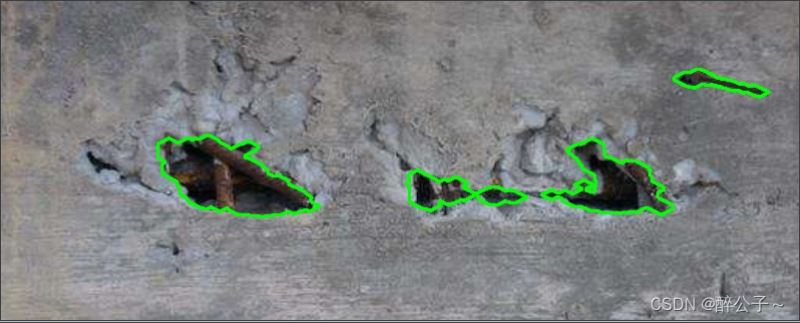﻿ Python+OpenCV实现表面缺陷检测_python_脚本之家

# Python+OpenCV实现表面缺陷检测

更新时间：2022年08月05日 15:13:00   作者：醉公子～• 将图像转化为二值图像
• 在对图像进行腐蚀/膨胀处理
• 在进行轮廓检测
• 筛选目标大小符合的轮廓（排除误差小的轮廓）
• 在在进行膨胀化处理，将轮廓信息绘制出
```import cv2
import os
import numpy as np
import time

t1 = time.time()
print(np.shape(img))
# 先利用二值化去除图片噪声
ret, img = cv2.threshold(img, 80, 255, cv2.THRESH_BINARY)

es = cv2.getStructuringElement(cv2.MORPH_ELLIPSE, (4, 2))
img = cv2.dilate(img, es, iterations=1)  # 形态学膨胀

kernel = np.ones(shape=[5,5],dtype=np.uint8)
img = cv2.erode(img,kernel=kernel)  # 腐蚀操作

cv2.imshow('aa',img)
cv2.waitKey(0)

contours, _ = cv2.findContours(img, cv2.RETR_TREE, cv2.CHAIN_APPROX_SIMPLE)

n = len(contours)  # 轮廓的个数
cv_contours = []
for contour in contours:
area = cv2.contourArea(contour)

if area <= 500:# 筛选面积大于500的，小于500的全部变为255，
cv_contours.append(contour)
# 方式一
# x, y, w, h = cv2.boundingRect(contour) # 这个函数可以获得一个图像的最小矩形边框一些信息，参数img是一个二值图像，它可以返回四个参数，左上角坐标，矩形的宽高 (轮廓集合  contour)
# img[y:y + h, x:x + w] = 255

else:

cv2.drawContours(img_copy, [contour], -1, (0, 0, 255), 0) # 多边形轮廓绘制

print('area:', area)
continue
# 方式二
cv2.fillPoly(img, cv_contours, (255, 255, 255)) # 多个多边形填充

t2 = time.time()
print('时间：',t2-t1)
cv2.imwrite('./output/28901647.jpg', img)
```

1、寻找到的轮廓信息（缺陷）2、通过腐蚀、膨胀后的，筛选出的较大缺陷3、通过不同程度的膨胀腐蚀、缺陷面积筛选